# Continuity equation

(diff) ← Older revision | Latest revision (diff) | Newer revision → (diff)
Jump to: navigation, search

One of the basic equations in hydrodynamics, expressing the law of conservation of mass for any volume of a moving fluid (or gas). In Euler variables the continuity equation has the form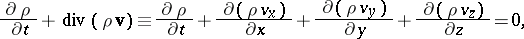whereis the density of the fluid,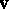is its velocity at a given point, and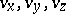are the projections of the velocity on the coordinate axes. If the fluid is incompressible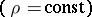, then the continuity equation takes the formFor a stationary one-dimensional flow in a tube, canal, etc., with cross-sectional area, the continuity equation gives the law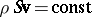for the flow.

How to Cite This Entry:
Continuity equation. Encyclopedia of Mathematics. URL: http://encyclopediaofmath.org/index.php?title=Continuity_equation&oldid=13303
This article was adapted from an original article by BSE-3 (originator), which appeared in Encyclopedia of Mathematics - ISBN 1402006098. See original article